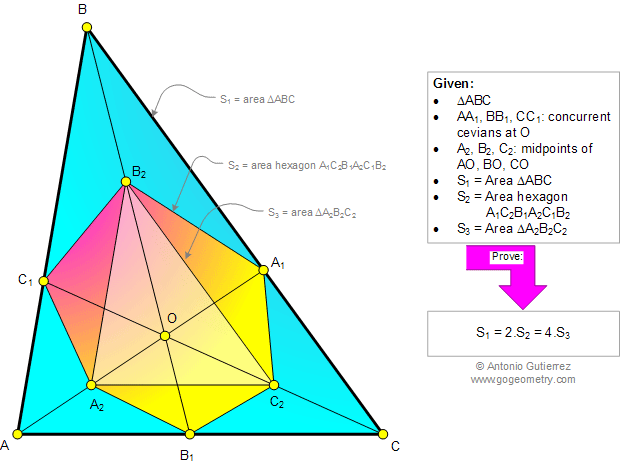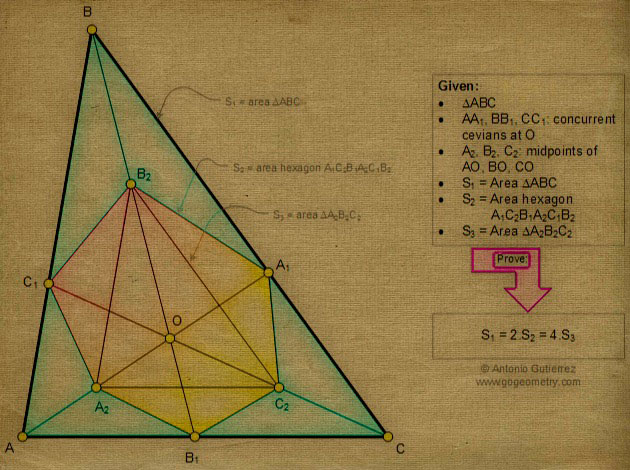# Geometry Problem 981. Triangle, Concurrent Cevians, Midpoints, Area, Hexagon

< PREVIOUS PROBLEM  |  NEXT PROBLEM >

AA1, BB1, and CC1 are concurrent cevians of triangle ABC at O (see the infographic below). A2, B2, and C2 are the midpoints of AO, BO, and CO, respectively. If S1 S2 and S3 are the areas of triangle ABC, hexagon A1C2B1A2C1B2, and triangle A2B2C2, prove that S1 = 2.S2 = 4.S3## Sketch of problem 981Geometry
Problems
All Problems
Open Problems
Visual Index
10 Problems
Problems Art Gallery
Art
981-990
Triangles
Triangle Area
Hexagon
Midpoints
Concurrency

Add or view a solution to the problem 981

Home | SearchGeometry